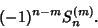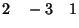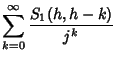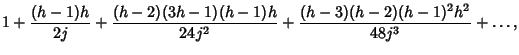## Stirling Number of the First Kind

The definition of the (signed) Stirling number of the first kind is a numbersuch that the number of permutations ofelements which contain exactlyCycles is(1)

This means thatforand. The Generating Function is(2)

This is the Stirling number of the first kind returned by the Mathematica(Wolfram Research, Champaign, IL) command StirlingS1[n,m]. The triangle of signed Stirling numbers of the first kind is(Sloane's A008275).

The Nonnegative version simply gives the number of Permutations ofobjects havingCycles (with cycles in opposite directions counted as distinct) and is obtained by taking the Absolute Value of the signed version. The nonnegative Stirling number of the first kind is denotedor. Diagrams illustrating,,, and(Dickau) are shown below.The nonnegative Stirling numbers of the first kind satisfy the curious identity(3)

(Gosper) and have the Generating Function(4)

and satisfy(5)

The Stirling numbers can be generalized to nonintegral arguments (a sort of Stirling polynomial'') using the identity(6)

which is a generalization of an Asymptotic Series for a ratio of Gamma Functions(Gosper).

See also Cycle (Permutation), Harmonic Number, Permutation, Stirling Number of the Second Kind

References

Abramowitz, M. and Stegun, C. A. (Eds.). Stirling Numbers of the First Kind.'' §24.1.3 in Handbook of Mathematical Functions with Formulas, Graphs, and Mathematical Tables, 9th printing. New York: Dover, p. 824, 1972.

Adamchik, V. On Stirling Numbers and Euler Sums.'' J. Comput. Appl. Math. 79, 119-130, 1997.

Conway, J. H. and Guy, R. K. In The Book of Numbers. New York: Springer-Verlag, pp. 91-92, 1996.

Dickau, R. M. Stirling Numbers of the First Kind.'' http://forum.swarthmore.edu/advanced/robertd/stirling1.html.

Knuth, D. E. Two Notes on Notation.'' Amer. Math. Monthly 99, 403-422, 1992.

Sloane, N. J. A. Sequence A008275 in The On-Line Version of the Encyclopedia of Integer Sequences.'' http://www.research.att.com/~njas/sequences/eisonline.html.